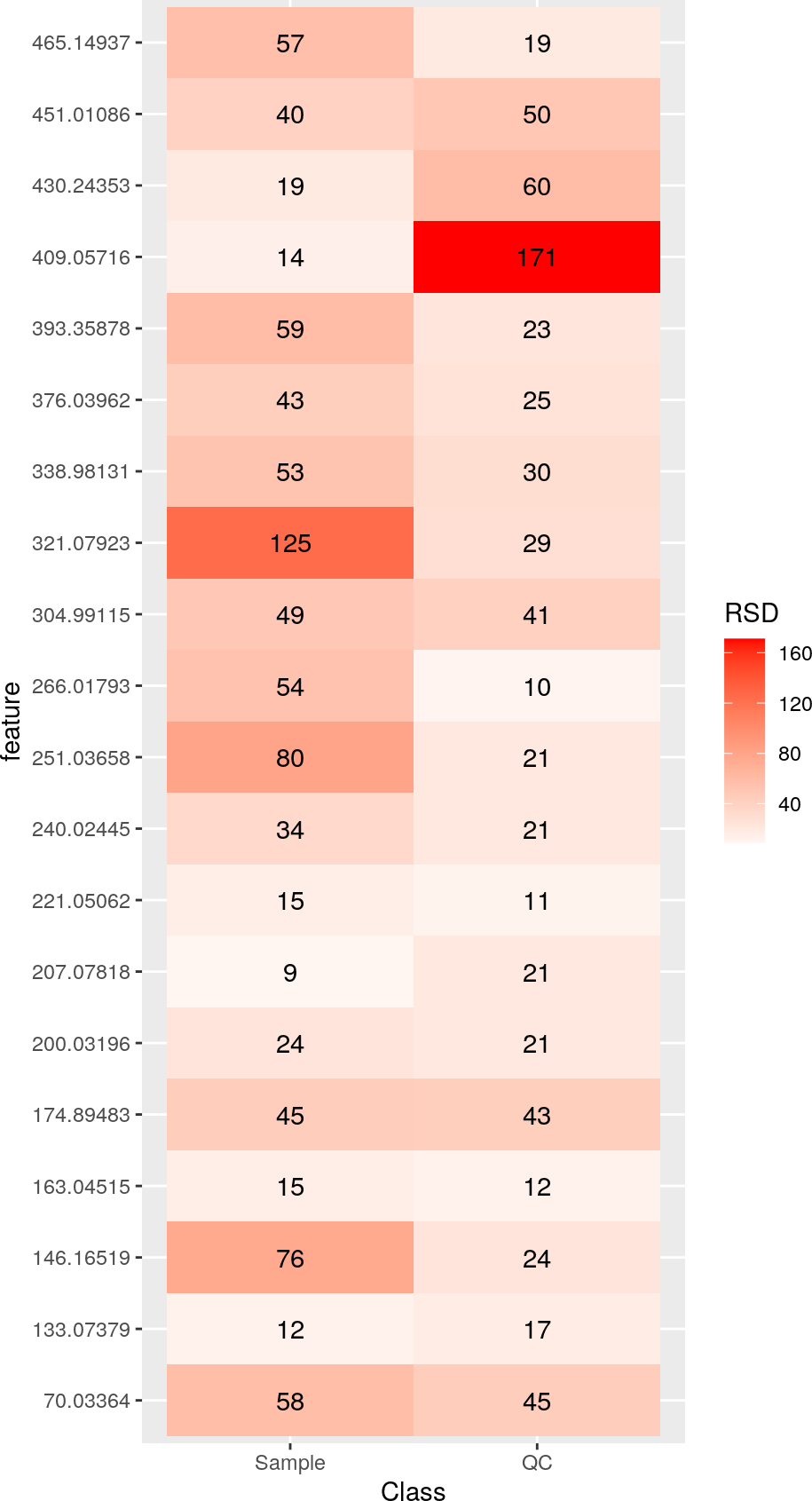# 1 Introduction

This is a step by step tutorial on how to assess and/or correct signal drift and batch effects within/across a multi-batch direct infusion mass spectrometry (DIMS) dataset. The same approach can be used on liquid chromatography mass spectrometry (LCMS) peak table as well.

Deeper details on how the algorithm works are detailed in 4.3

# 2 Installation

You should have R version 4.0.0 or above and Rstudio installed to be able to run this notebook.

Execute following commands from the R terminal.

``````install.packages("gridExtra")

if (!requireNamespace("BiocManager", quietly = TRUE))
install.packages("BiocManager")
BiocManager::install("pmp")``````

Load the required libraries into the R environment

``````library(S4Vectors)
library(SummarizedExperiment)
library(pmp)
library(ggplot2)
library(reshape2)
library(gridExtra)``````

# 3 Data set

In this tutorial we will be using an direct infusion mass spectrometry (DIMS) data set consisting of 172 samples measured across 8 batches and is included in `pmp` package as `SummarizedExperiemnt` class object `MTBLS79`. More detailed description of the data set is available from Kirwan et al. (2014), MTBLS79 and R man page.

``help ("MTBLS79")``
``````feature_names <- c("70.03364", "133.07379", "146.16519", "163.04515",
"174.89483", "200.03196", "207.07818", "221.05062", "240.02445",
"251.03658", "266.01793", "304.99115", "321.07923", "338.98131",
"376.03962", "393.35878", "409.05716", "430.24353", "451.01086",
"465.14937")
summary(t(SummarizedExperiment::assay(MTBLS79[feature_names, ])))
#>     70.03364       133.07379        146.16519        163.04515
#>  Min.   : 2390   Min.   :214680   Min.   : 20142   Min.   : 85138
#>  1st Qu.:15107   1st Qu.:326793   1st Qu.: 43129   1st Qu.:140682
#>  Median :25992   Median :364517   Median : 57957   Median :153265
#>  Mean   :25715   Mean   :355800   Mean   : 73911   Mean   :154682
#>  3rd Qu.:35395   3rd Qu.:389726   3rd Qu.: 85819   3rd Qu.:170635
#>  Max.   :56061   Max.   :452978   Max.   :351751   Max.   :216917
#>  NA's   :18
#>    174.89483       200.03196       207.07818        221.05062
#>  Min.   : 6839   Min.   :18338   Min.   :179175   Min.   : 52204
#>  1st Qu.:11551   1st Qu.:24673   1st Qu.:206967   1st Qu.: 86777
#>  Median :14079   Median :28380   Median :220686   Median : 96552
#>  Mean   :16982   Mean   :30027   Mean   :225574   Mean   : 96794
#>  3rd Qu.:21159   3rd Qu.:33026   3rd Qu.:234812   3rd Qu.:105478
#>  Max.   :43762   Max.   :58147   Max.   :431784   Max.   :131368
#>
#>    240.02445       251.03658        266.01793       304.99115
#>  Min.   :12994   Min.   :  4726   Min.   : 5283   Min.   : 24683
#>  1st Qu.:22179   1st Qu.: 20658   1st Qu.:14247   1st Qu.: 44669
#>  Median :28939   Median : 30675   Median :30224   Median : 60338
#>  Mean   :29429   Mean   : 41251   Mean   :26680   Mean   : 68689
#>  3rd Qu.:34527   3rd Qu.: 58220   3rd Qu.:36028   3rd Qu.: 80126
#>  Max.   :54417   Max.   :164754   Max.   :61402   Max.   :190193
#>                  NA's   :32       NA's   :30
#>    321.07923        338.98131       376.03962       393.35878
#>  Min.   :  5368   Min.   : 2817   Min.   : 7082   Min.   : 62973
#>  1st Qu.: 16107   1st Qu.: 6413   1st Qu.:17636   1st Qu.:123464
#>  Median : 27822   Median : 8215   Median :22720   Median :166579
#>  Mean   : 38301   Mean   : 9494   Mean   :24130   Mean   :235819
#>  3rd Qu.: 46408   3rd Qu.:11087   3rd Qu.:28685   3rd Qu.:334867
#>  Max.   :285756   Max.   :30462   Max.   :67877   Max.   :830668
#>  NA's   :27       NA's   :29
#>    409.05716         430.24353        451.01086       465.14937
#>  Min.   : 110970   Min.   : 25415   Min.   : 1884   Min.   :  3167
#>  1st Qu.: 151404   1st Qu.: 34350   1st Qu.: 5254   1st Qu.: 19916
#>  Median : 161617   Median : 38528   Median : 7210   Median : 25224
#>  Mean   : 183870   Mean   : 43203   Mean   : 7880   Mean   : 27209
#>  3rd Qu.: 174052   3rd Qu.: 43726   3rd Qu.:10490   3rd Qu.: 29656
#>  Max.   :2828059   Max.   :121995   Max.   :17602   Max.   :101471
#>                                     NA's   :1       NA's   :9``````
``````#number of samples:
ncol(MTBLS79)
#>  172``````
``````#Batches:
unique(MTBLS79\$Batch)
#>  "1" "2" "3" "4" "5" "6" "7" "8"``````
``````#Sample classes:
unique(MTBLS79\$Class)
#>  "QC" "C"  "S"``````

# 4 Explorative example

A more detailed overview and guidelines on strategies for quality control of mass spectrometry assays is detailed in recent work by Broadhurst et al. (2018).

To evaluate if the data needs correction, it is common practice to examine the relative standard deviation (RSD) of the quality control (QC) samples and biological samples. RSD% is also sometimes referred to as the coefficient of variation (CV). An RSD% for the QC samples below 20-30% is commonly used as an acceptable level of technical variation where signal correction is not required.

The following code calculates and plots the RSD% values of the features within the data set.

``````#  separate the LCMS data from the meta data
data(MTBLS79)
data <- SummarizedExperiment::assay(MTBLS79[feature_names, ])
class <- SummarizedExperiment::colData(MTBLS79)\$Class
batch <- SummarizedExperiment::colData(MTBLS79)\$Batch
order <- c(1:ncol(data))

# get index of QC samples
QChits <- which(class == "QC")

# small function to calculate RSD%
FUN <- function(x) sd(x, na.rm=TRUE) / mean(x, na.rm=TRUE) * 100

# RSD% of biological and QC samples within all 8 batches:
out <- matrix(ncol=2, nrow=nrow(data))
colnames(out) <- c("Sample","QC")
rownames(out) <- rownames(data)

# for each feature calculate RSD% for the samples and the QCs
for (i in 1:nrow(data)) {
out[i, 1] <- FUN(data[i, -QChits]) # for samples
out[i, 2] <- FUN(data[i, QChits]) # for QCs
}

# prepare data for plotting
plotdata <- melt(data.frame(out), variable.name="Class", value.name="RSD")
plotdata\$feature <- rownames(data)

plotdata\$RSD <- round(plotdata\$RSD,0)
plotdata\$feature <- factor(plotdata\$feature, ordered=TRUE,
levels=unique(plotdata\$feature))

# plot
ggplot(data=plotdata, aes(x=Class, y=feature, fill=RSD)) +
geom_tile() +
geom_text(aes(label=RSD)) +``````ggplot(data=plotdata, aes(x=Class, y=RSD, fill=Class)) +